# Problem 2.4 Griffiths E&M 3rd ed -- E-field above a square loop

## Homework Statement

The problem states, "Find the electric field a distance z above the center of a square loop (sides of length a) carrying a uniform line charge λ. " The hint says to use the result of example 2.1.

Example 2.1 is a similar problem, but instead of a square loop you are asked to "Find the electric field a distance z above the midpoint of a straight line segment of length 2L which carries a uniform line charge λ."

## Homework Equations

The electric field of a line charge: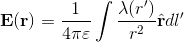(equation 1)

The example starts by putting this equation (in the form of dE) into the z-direction by putting a cosθ at the end. It looks like this: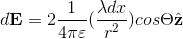(equation 2)

The problem is solved and this result is given: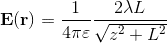(equation 3)
The example states that this equation is in the z-direction, and this is the equation that will be used for problem 2.4.

## The Attempt at a Solution

I am following the solutions manual on this one, as I am doing all of this for self-study. There is just one thing that I cannot understand from the solution.

To adapt the above equation to problem 2.4, we make the substitution that L=a/2 , or L=2a.
Then substitute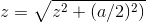This results in: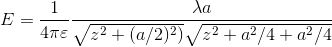Then multiply by 4 because we have a square loop instead of just one line segment.

Here is what I do not understand: the solutions manual states to now multiply by cosθ to get only the z-direction. But wasn't that already done to derive equation 3 that we used for this problem? The book even states that this equation is in the z-direction. So why do it again??

I hope this is enough information, thanks for your help!

TSny
Homework Helper
Gold Member
Here is what I do not understand: the solutions manual states to now multiply by cosθ to get only the z-direction. But wasn't that already done to derive equation 3 that we used for this problem? The book even states that this equation is in the z-direction. So why do it again??

Hello, and welcome to PF!

Think about the direction of the electric field due to one side of the square at the point that is located a distance z above the midpoint of the square.

Aside: Logically speaking, the solution manual should have first multiplied by ##\cos \theta## and then multiplied by 4.